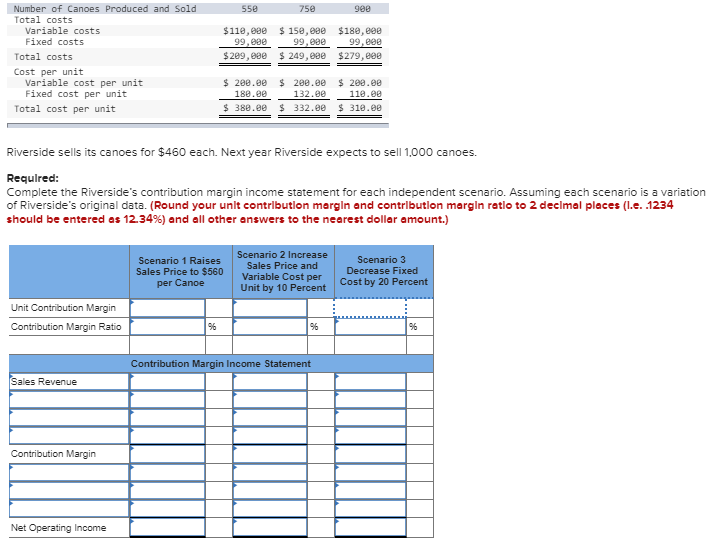### Create an Account

Home / Questions / Number of Canoes Produced and Sold 550 75e 900 Total costs Variable Costs \$110,08e 99.00e 150,00e 99

# Number of Canoes Produced and Sold 550 75e 900 Total costs Variable Costs \$110,08e 99.00e 150,00e 99Number of Canoes Produced and Sold 550 75e 900 Total costs Variable Costs \$110,08e 99.00e 150,00e 99,08e \$180,eee 99,eee Fixed costs 249,08e \$209,0e \$279,eee Total costs Cost per unit Variable cost per unit Fixed cost per unit \$ 200.0e \$ 208.0e 208.e 180.e0 132.00 118.0e \$ 380.00 \$ 318.00 Total cost per unit \$ 332.0e Riverside sells its canoes for \$460 each. Next year Riverside expects to sell 1,000 canoes. Required: Complete the Riverside&#39;s contribution margin income statement for each independent scenario. Assuming each scenario is a variation of Riverside&#39;s original data. (Round your unlt contributlon margin and contributlon margin reatio to 2 decimal places (l.e. .1234 should be entered as 12.34%) and all other answers to the nearest dollar amount.) Scenario 2 Increase Scenario 3 Scenario 1 Raises Sales Price and Sales Price to \$560 per Canoe Decrease Fixed Variable Cost per Unit by 10 Percent Cost by 20 Percent Unit Contribution Margin % Contribution Margin Ratio % % Contribution Margin Income Statement Sales Revenue Contribution Margin Net Operating Income

Apr 04 2020 View more View LessSubscribe To Get Solution